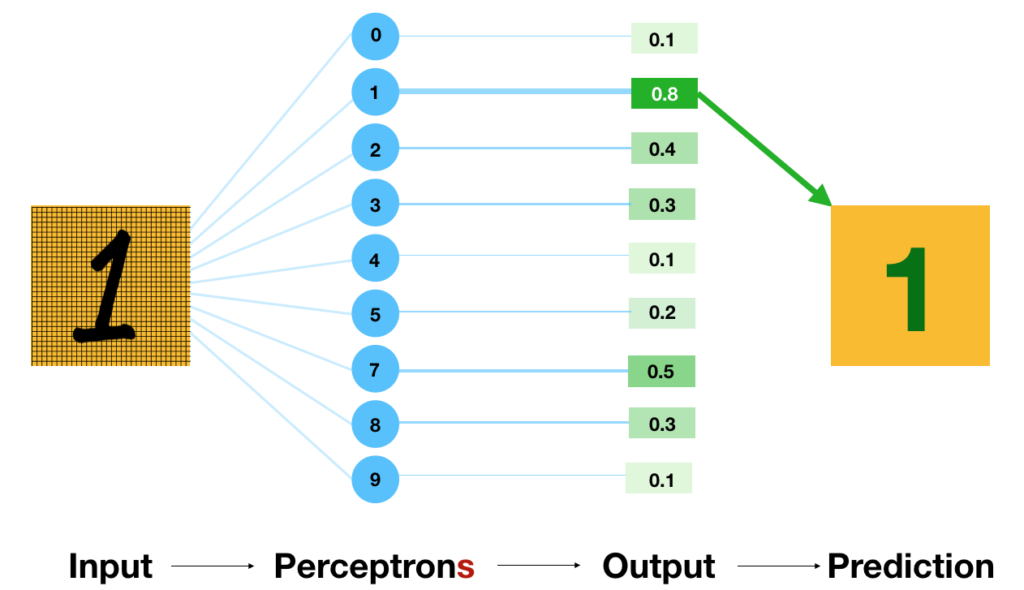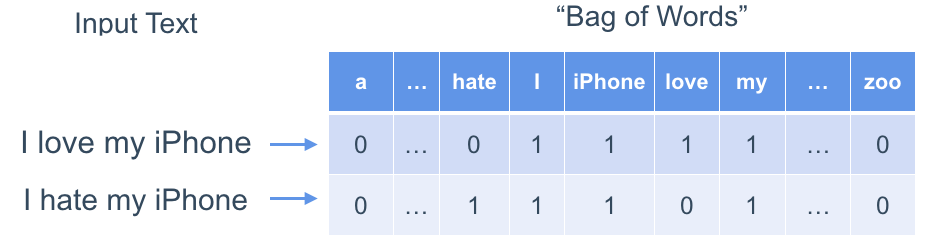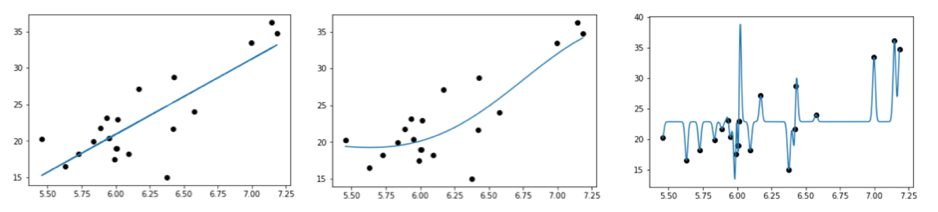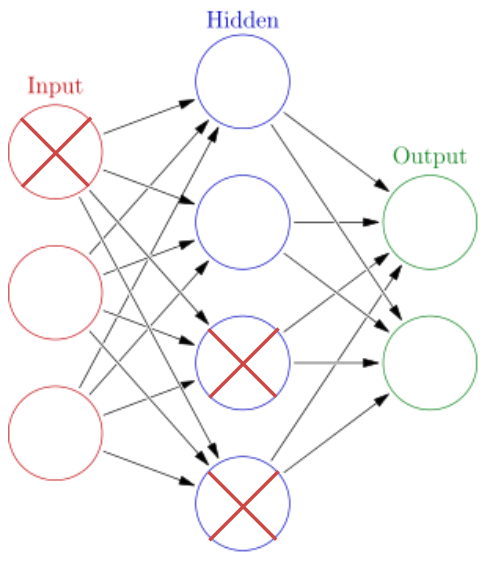## Multilayer Perceptrons

In this video we build a more complicated network for classifying images and cover some details of how neural networks function.

Project

Check your understanding by building a fashion classifier by going to https://github.com/lukas/ml-class/tree/master/projects/2-fashion-mnist-mlp

Topics

• One hot encoding
• New loss functions: categorical cross-entropy
• New activation functions: ReLU
• Data normalization
• Using dropout to reduce overfitting

## Multilayer Perceptrons

Now that we know how to create a perceptron that classifies fives based on whether the output is a 0 or 1, how should we expand our model to recognize all digits 0-9?

The answer, which may be surprising, is to have 10 perceptrons running in parallel, where each perceptron is responsible for a digit. For instance, we have a perceptron responsible for classifying zeros, another for ones, another for twos etc. For an image, we will predict the digit based on whichever perceptron outputs the highest number. For example, if input the handwritten image of a 7, we would like ‘perceptron 7’ to output a number really close to 1, and for all the other perceptrons to output 0.Our loss function for a multiclass perceptrons, is the difference between the target – the ideal output we would like to receive for each perceptron – and our actual output.## One Hot Encoding

The ideal output we would like if given a number 3, would be ‘001000000’: the perceptron for recognising threes outputs a 1 and every other perceptron outputs a 0. In order to transform our output labels (3) into that format (00100000), we use one-hot encoding.One-hot encoding is a very common practice and transforms a number into a binary array. In the code, we can see this on lines 20-23 in perceptron-linear.py. We convert both our training data labels and our test data labels into one hot encodings that we can compare with our output.

## Activation Functions

After running our model, we get around a 16% accuracy on the run (not deterministic so you may get a different number). In machine learning, you want to first consider what accuracy random guessing would get. There’s 10 digits and our data is evenly distributed so random guessing would give us around 10% accuracy.

We’re off to a good start but we can improve this model.  The first thing to do is to fix the activation function.  Remember that our target values are ones and zeros, but our weighted sum can be anything. This means that we want a way to convert that weighted sum into a number between 0 and 1.

To do this, we can use an activation function. A sigmoid or softmax activation function will take the weighted sum and squeeze the output to a number between zero and one. You always want to use a softmax activation function on your last layer of your network if you are doing classification, to constrain your output to be between zero and one. The code including the activation function is in perceptron-logistic.py.

This makes our model work significantly better, but there are still improvements we can make.

## Categorical Cross Entropy

In the first video, we introduced the idea of loss functions. We saw the difference between absolute error and mean squared error, and in what circumstances you should use each. When categorizing things however, you want to use the loss function categorical cross entropy.Categorical cross entropy loss gets neural nets to output their true probability of an outcome.  If the answer you are looking for is 1 categorical cross entropy will give you an infinite loss if you predict 0 and diminishing returns the closer you predict to 1 (remember with loss functions lower is better). This means the model will never output a probability of zero unless it is 100% sure it wasn’t the right answer.

This improves things quite a bit, and now if we want to improve our accuracy we can turn to our data.

## Normalizing Data

Neural networks are not scale-invariant. Most neural network libraries really care about your input range, and you get much better results from normalizing your data to be between 0 and 1. In our case, our input is pixel values, which is between 0 and 255. To convert all of these pixel values to a number between 0 and 1 (normalize our data), we divide every value by 255. The complete code is in perceptron-normalize.py.

This change helps a lot – our accuracy increases from 15% to 90%!!

## Debugging Summary

Here we have seen some general techniques to debug a machine learning model. Firstly, add a softmax activation function to convert the weighted sum to a number between 0 and 1. Secondly, change the loss function to categorical cross entropy to output the true probability of each category. Thirdly, clean up and normalize training data.

## Multilayer Perceptrons

We now have one layer of perceptrons working well, but how can we further optimize our model? Currently, our model only captures the relationships between individual pixels and a label. To make our model recognize interactions between pixels, we need to add some layers to our perceptron.

Multilayer perceptrons take the output of one layer of perceptrons, and uses it as input to another layer of perceptrons. This creates a “hidden layer” of perceptrons in between the input layer and the output layer. This hidden layer works the same as the output layer, but instead of classifying, they just output numbers.

Typically hidden layers use an activation function called ReLU. The Rectified Linear Unit (ReLU) activation function simply truncates negative values. This linear function is really fast, and so works great for hidden layers.Open up mlp.py. This code is very similar to our perceptron model with just a single line added on line 40. Now we have a second dense layer with a number of hidden nodes.  config.hidden_nodes is set to 100 but you can try other numbers.

Config.hidden_nodes = 100
//line 40

When you run the model now, you may notice that the accuracy rises above the validation accuracy. Overtime, the accuracy will continue to improve, whereas the validation accuracy will remain the same. This phenomena is known as overfitting.

## Overfitting & DropoutOverfitting occurs when the model learns the training data with too much specificity, and cannot generalize well to new input. We can tell our model is overfitting when the training data accuracy is higher than the validation accuracy. Since validation accuracy assesses the performance of the model on data that it has not already seen, it is a better indicator of a models’ performance than plain accuracy.

Overfitting is a huge problem for machine learning, since in order to improve our model we may increase the complexity, but this only worsens overfitting. As a general rule of thumb, if your model is overfitting, then anything you do to make your model fancier will only hurt you – you have to fix the overfitting first. Luckily, there is an easy to use algorithm which really helps reduce overfitting: dropout.The solution is to set some fraction of the inputs (in this case, pixel values) to 0. Why would this help?  Dropout forces the network to learn more than one reason for every classification.  Imagine a pixel in the upper right was always lit up if and only if the digit was a 7.  Our network would happily learn that it only needs to look at the upper left hand digit to decide if the handwritten number is a 7 to optimize loss on the training data.  Dropout would force the network to learn multiple pathways for deciding if a digit is 7 because some fraction of the time that value would be hidden.

We’ve debugged our multilayer and multiclass perceptron and really improved the accuracy by dealing with common issues like data normalization and overfitting. But we still haven’t squeezed the highest possible accuracy out of this classic dataset. In the next tutorial we’ll check out convolutional neural networks.